RRB Group D Mock Test - 30

# RRB Group D Mock Test - 30

Test Description

## 100 Questions MCQ Test RRB (Group D) - Mock Tests & Previous Year Papers | RRB Group D Mock Test - 30

RRB Group D Mock Test - 30 for Railways 2022 is part of RRB (Group D) - Mock Tests & Previous Year Papers preparation. The RRB Group D Mock Test - 30 questions and answers have been prepared according to the Railways exam syllabus.The RRB Group D Mock Test - 30 MCQs are made for Railways 2022 Exam. Find important definitions, questions, notes, meanings, examples, exercises, MCQs and online tests for RRB Group D Mock Test - 30 below.
Solutions of RRB Group D Mock Test - 30 questions in English are available as part of our RRB (Group D) - Mock Tests & Previous Year Papers for Railways & RRB Group D Mock Test - 30 solutions in Hindi for RRB (Group D) - Mock Tests & Previous Year Papers course. Download more important topics, notes, lectures and mock test series for Railways Exam by signing up for free. Attempt RRB Group D Mock Test - 30 | 100 questions in 90 minutes | Mock test for Railways preparation | Free important questions MCQ to study RRB (Group D) - Mock Tests & Previous Year Papers for Railways Exam | Download free PDF with solutions
 1 Crore+ students have signed up on EduRev. Have you?
RRB Group D Mock Test - 30 - Question 1

### The speaker of Lok Sabha addresses his later of resignation to the?

RRB Group D Mock Test - 30 - Question 2

### Asteroid Belt which consists of numerous Irregularly shaped bodies called Asteroids or Minor planets is observed between the orbits of which of the following Planets ?

RRB Group D Mock Test - 30 - Question 3

### The first leader to use the word ”Swaraj” was?

RRB Group D Mock Test - 30 - Question 4

Which of the following countries use Peso as their currency?

RRB Group D Mock Test - 30 - Question 5

What is the strength of legislative assembly in Delhi?

RRB Group D Mock Test - 30 - Question 6

When did Delhi become a union territory?

RRB Group D Mock Test - 30 - Question 7

With which part of the body is the disease "Bronchitis" associated?

RRB Group D Mock Test - 30 - Question 8

Who built the Jagannatha temple of Puri ?

RRB Group D Mock Test - 30 - Question 9

Ultra purification of metal is done by?

RRB Group D Mock Test - 30 - Question 10

Who has won the 2017 Nobel Prize in Economics?

RRB Group D Mock Test - 30 - Question 11

Who has become the first Indian to win an International Medal - Bronze in Skiing recently in the Alpine Ejder 3200 Cup held in January?

RRB Group D Mock Test - 30 - Question 12

Which country topped the Commonwealth Games 2014 Medal tally ?

RRB Group D Mock Test - 30 - Question 13

Which of the following countries is not a member of the G-7 Countries?

RRB Group D Mock Test - 30 - Question 14

Which of the following countries is not a member of the OPEC Countries?

RRB Group D Mock Test - 30 - Question 15

Guru Tej Bahadur trophy is related to which sport?

RRB Group D Mock Test - 30 - Question 16

What is the Venue of next FIFA 2018 World cup?

RRB Group D Mock Test - 30 - Question 17

What is the full form of USB as related to computers?

RRB Group D Mock Test - 30 - Question 18

Which Indian city has the largest cricket stadium based on the seating capacity?

RRB Group D Mock Test - 30 - Question 19

Who was the chairman of the Drafting Committee of the Constitution of India?

RRB Group D Mock Test - 30 - Question 20

Who assassinated Mahatma Gandhi?

RRB Group D Mock Test - 30 - Question 21

Ministry of Railways has launched which of the following applications to help Freight Managers to monitor and manage Freight business using Geographic Information System views and Dashboard?

RRB Group D Mock Test - 30 - Question 22

Ministry of Railways has set a target for Installing Bio- Toilets in all trains in India by which of the following dates?

RRB Group D Mock Test - 30 - Question 23

Who is the current Prime Minister of U.K. ?

RRB Group D Mock Test - 30 - Question 24

Who is sumitra mahajan?

RRB Group D Mock Test - 30 - Question 25

Who was the Governor General of India when the first war of Independence broke out in 1857?

RRB Group D Mock Test - 30 - Question 26

Who is the richest player in FIFA (According to Business Insider) ?

RRB Group D Mock Test - 30 - Question 27

The Andes mountains are located in which continent?

RRB Group D Mock Test - 30 - Question 28

Indian Railways has recently announced to equip 8500 Stations with Wifi by which of the following dates?

RRB Group D Mock Test - 30 - Question 29

Which of the following countries is a part of the European Union?

RRB Group D Mock Test - 30 - Question 30

Which of the following is the correct Abbreviation of TCPO?

RRB Group D Mock Test - 30 - Question 31

Who invented electric battery?

RRB Group D Mock Test - 30 - Question 32

When a person tries to stretch a cable, the force that pulls the person in the opposite direction is called ….

RRB Group D Mock Test - 30 - Question 33

Lack of vitamin B complex causes

RRB Group D Mock Test - 30 - Question 34

When the surface of a hot object can not be accessed, we use specialised thermometers to measure the temperature. Such thermometers are called ….

RRB Group D Mock Test - 30 - Question 35

In which year did Indira Gandhi impose an emergency in India quoting “internal disturbance”?

RRB Group D Mock Test - 30 - Question 36

Which of the following states has no world heritage sites as per UNESCO?

RRB Group D Mock Test - 30 - Question 37

An altimeter measures the height above sea level by measuring …. of the ambience.

RRB Group D Mock Test - 30 - Question 38

Who was the last Governor General of India?

RRB Group D Mock Test - 30 - Question 39

With which state is Kathakali dance form associated with?Report Question

RRB Group D Mock Test - 30 - Question 40

What is the birthplace of Mahatma Gandhi?

RRB Group D Mock Test - 30 - Question 41

What is the name of tiger reserve Present in Maharashtra?

RRB Group D Mock Test - 30 - Question 42

Which state has the highest per capita milk availability?

RRB Group D Mock Test - 30 - Question 43

In which year did Sikkim join the Indian Republic?

RRB Group D Mock Test - 30 - Question 44

"Night blindness" is caused due to deficiency of which vitamin?

RRB Group D Mock Test - 30 - Question 45

Which strong acid is commonly called 'Oil of Vitriol'?

RRB Group D Mock Test - 30 - Question 46

Who was the first President of Republic of India?

RRB Group D Mock Test - 30 - Question 47

Find the odd man out among the options:

Detailed Solution for RRB Group D Mock Test - 30 - Question 47

Other than Ujjain, all the other three are names of the same city.

RRB Group D Mock Test - 30 - Question 48

Filariasis is a disease that generally spreads through ….

RRB Group D Mock Test - 30 - Question 49

Solar Panels convert … energy to … energy

RRB Group D Mock Test - 30 - Question 50

When Potassium Permanganate is added to a jar containing hydrogen peroxide, oxygen is released. In this reaction potassium permanganate acts as a/an …

RRB Group D Mock Test - 30 - Question 51

Which of the following countries is not a member of the SCO - Shanghai Cooperation Organisation?

RRB Group D Mock Test - 30 - Question 52

Where is the largest shipyard located in India?

RRB Group D Mock Test - 30 - Question 53

Which of the following is a NON renewable source of energy?

RRB Group D Mock Test - 30 - Question 54

Which of the following is the lightest metal?

RRB Group D Mock Test - 30 - Question 55

Which one of the following is the best conductor of electricity?

RRB Group D Mock Test - 30 - Question 56

Which of the following is the study and Interpretation of Ancient Inscriptions/Writings?

RRB Group D Mock Test - 30 - Question 57

The term olfactory relates to the sense of ….

RRB Group D Mock Test - 30 - Question 58

When a star dies, there is usually a huge explosion. What is it called?

RRB Group D Mock Test - 30 - Question 59

Who is recently appointed as the next Chairman of ICHR - Indian Council of Historical Research?

RRB Group D Mock Test - 30 - Question 60

Sound waves are a classic example of

RRB Group D Mock Test - 30 - Question 61

A is 20% more efficient than B. B can complete a piece of in 20 days. In how many days can A do the same work (Approx)?

Detailed Solution for RRB Group D Mock Test - 30 - Question 61

Let efficiency of B = 100%

Efficiency of A = 120%

120(x) = 100(20)

x = 100/6 = 16.66 = 17 full days

So the answer is option D.

RRB Group D Mock Test - 30 - Question 62

A bus travels 600kms in 15hrs and another 300 kms in 10 hrs.Find the average speed ?

Detailed Solution for RRB Group D Mock Test - 30 - Question 62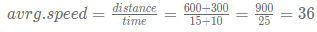So the answer is option B.

RRB Group D Mock Test - 30 - Question 63

A Train 280 metre long speeds past a man in 20 seconds. What is its speed in kmph ?

Detailed Solution for RRB Group D Mock Test - 30 - Question 63

Speed = distance/time = 280/20 = 14 m/s = 14*(18/5) = 50.4 kmph

So the answer is option A.

RRB Group D Mock Test - 30 - Question 64

An alloy of gold and silver weighs 50 gms. It contains 80% gold. How much gold should be added to increase the percentage of gold in the alloy to 90% ?

Detailed Solution for RRB Group D Mock Test - 30 - Question 64

alloy weight = 50g

gold = 80% of 50 = 40g & silver = 10g

If we add 50grams of gold to 50g alloy, alloy becomes 100g

So in 100g of alloy, gold = 40+50 = 90g and silver = 10g

Now gold is 90% in the alloy

So the answer is option C.

RRB Group D Mock Test - 30 - Question 65

What is the difference between the LCM and HCF of 24, 48 ?

Detailed Solution for RRB Group D Mock Test - 30 - Question 65

LCM of 24 and 48 = 48

HCF of 24 and 48 = 24

Difference = 24

So the answer is option D.

RRB Group D Mock Test - 30 - Question 66

Find the mode of 2,3,4, 2,2,3,2,3,4,5,3,5,3,2,5,2 ?

Detailed Solution for RRB Group D Mock Test - 30 - Question 66

Mode is the number which occurred frequently = 2

So the answer is option A.

RRB Group D Mock Test - 30 - Question 67

3245 - 123 - 456 - 347 + x = 3650 ?

Detailed Solution for RRB Group D Mock Test - 30 - Question 67

3245 - 123 - 456 - 347 + x = 3650

2319 + x = 3650

x = 1331

So the answer is option B.

RRB Group D Mock Test - 30 - Question 68

Which of the following is divisible by 3, 7, 9 and 11 ?

Detailed Solution for RRB Group D Mock Test - 30 - Question 68

3*7*9*11 = 2079

2079*2 = 4158 is divisible by 3, 7, 9, 11

So the answer is option B.

RRB Group D Mock Test - 30 - Question 69

The cost of 20 pens and 24 pencils is 256. What is the cost of 5 pens and 6 pencils ?

Detailed Solution for RRB Group D Mock Test - 30 - Question 69

The cost of 20 pens and 24 pencils is 256

20x+24y = 256

divide with 4

5x+6y = 64

So the cost of 5 pens and 6 pencils is 64.

So the answer is option C.

RRB Group D Mock Test - 30 - Question 70

Find the compound interest on 40000 at 6% per annum for 2 years compounded half yearly ?

Detailed Solution for RRB Group D Mock Test - 30 - Question 70

Total amount = P(1+\frac{r}{200})^{2n} = 40000(1+\frac{6}{200})^4 = 45020P(1+200r​)2n=40000(1+2006​)4=45020

CI = 45020 - 40000 = 5020

So the answer is option A.

RRB Group D Mock Test - 30 - Question 71

There are some sheep and hens. If there are a total of 36 legs and there are 14 animals and birds in total, how many sheep are there ?

Detailed Solution for RRB Group D Mock Test - 30 - Question 71

A sheep has 4 legs and hen has 2 legs

4x+2y = 36 ===> 2x+y = 18----(1)

x+y = 14 ----(2)

(1)-(2) ==> 2x+y-x-y = 18-14 = 4

So the answer is option A.

RRB Group D Mock Test - 30 - Question 72

FInd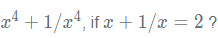Detailed Solution for RRB Group D Mock Test - 30 - Question 72

x+1/x = 2x+1/x=2

squaring on both sides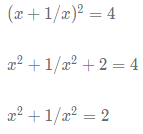squaring on both sides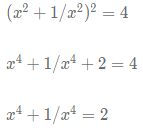So the answer is option D.

RRB Group D Mock Test - 30 - Question 73

Find the curved surface area of cone of radius 21cm and slant height 42cm ?

Detailed Solution for RRB Group D Mock Test - 30 - Question 73

Curved surface area of cone = \pi rl = (22/7)(21)(42) = 2772πrl=(22/7)(21)(42)=2772 sq.cm

So the answer is option C.

RRB Group D Mock Test - 30 - Question 74

Find the value of x, if 2x+3y = 4 and 4x+6y = 8 ?

Detailed Solution for RRB Group D Mock Test - 30 - Question 74

(4x+6y=8) is 2*(2x+3y=4)

So both represents the same equation, hence we cant find x or y

So the answer is option D.

RRB Group D Mock Test - 30 - Question 75

The ratio of the ages of Kohli and his wife is 7:6 while the sum of their ages after 4 years is 60. What is the age of Kohli?

Detailed Solution for RRB Group D Mock Test - 30 - Question 75

Let age of kohli = 7x

Age of his wife = 6x

Sum of their ages after 4 years = 60

7x+4 + 6x+4 = 60

13x = 52

x = 4

Age of kohli = 7x = 28

So the answer is option B.

RRB Group D Mock Test - 30 - Question 76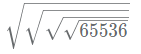?

Detailed Solution for RRB Group D Mock Test - 30 - Question 76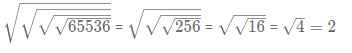So the answer is option A.

RRB Group D Mock Test - 30 - Question 77

At what time the hands of the clock show a mirror image of 4:10 ?

Detailed Solution for RRB Group D Mock Test - 30 - Question 77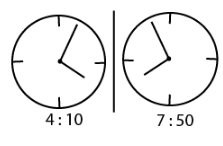It should be 7:50

So the answer is option C.

RRB Group D Mock Test - 30 - Question 78

Find 40% of 30% of 1200% of 20 ?

Detailed Solution for RRB Group D Mock Test - 30 - Question 78

40% of 30% of 1200% of 20 = (0.4)(0.3)(12)(20) = (0.12)(240) = 28.8

So the answer is option D.

RRB Group D Mock Test - 30 - Question 79

FInd the profit earned by a merchant if he sells 2 items worth 200/- each for 12% profit ?

Detailed Solution for RRB Group D Mock Test - 30 - Question 79

Profit earned on each item = 12% of 200 = 24/-

Total profit = 24(2) = 48/-

So the answer is option B.

RRB Group D Mock Test - 30 - Question 80

Two pipes A and B can fill a tank in 4 hrs and 8 hrs respectively. Both the pipes are opened simultaneously, find the time needed to fill the tank?

Detailed Solution for RRB Group D Mock Test - 30 - Question 80

Let Total capacity of tank = LCM of 4 and 8 = 8

Efficiency of A = 8/4 = 2

Efficiency of B = 8/8 = 1

Tank can be filled by both pipes in 8/(2+1) hrs = 8/3 hrs =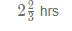So the answer is option C.

RRB Group D Mock Test - 30 - Question 81

Find the incorrect term in the sequence below - 48, 72, 108, 162, 244

Detailed Solution for RRB Group D Mock Test - 30 - Question 81

48 * 1.5 = 72
72 * 1.5 = 108
108 * 1.5 = 162
162 * 1.5 = 243
Hence, option C is the right answer.

RRB Group D Mock Test - 30 - Question 82

Find the incorrect term in the sequence below - 27, 46, 66, 81, 97, 111

Detailed Solution for RRB Group D Mock Test - 30 - Question 82

27 + 19 = 46
46 + 18 = 64
64 + 17 = 81
81 + 16 = 97
97 + 15 = 111
Hence, option B is the right answer.

RRB Group D Mock Test - 30 - Question 83

Find the incorrect term in the sequence below - 18, 29, 42, 57, 76, 93

Detailed Solution for RRB Group D Mock Test - 30 - Question 83

18 + 11 = 29
29 + 13 = 42
42 + 15 = 57
57 + 17 = 74
74 + 19 = 93
Hence, option A is the right answer.

Study the following information carefully and answer the questions which follow:
In a certain language
‘game water key chair’ is coded as ‘gn te di bo’
’chair moon bat water’ is coded as ‘pc di lz gn’
‘lake water key bat’ is coded as ‘lz bo gn hy’

RRB Group D Mock Test - 30 - Question 84

What is the code for ‘moon’?

Detailed Solution for RRB Group D Mock Test - 30 - Question 84

We know that,
‘game water key chair’ is coded as ‘gn te di bo’
’chair moon bat water’ is coded as ‘pc di lz gn’
‘lake water key bat’ is coded as ‘lz bo gn hy’
From 1st and 2nd we can say that the code for water and chair must be either gn or di. So the code for game and key must be either te or bo. From the third statement, we can say that code for lake is hy, code for key is bo and code for bat is lz.
Thus we have,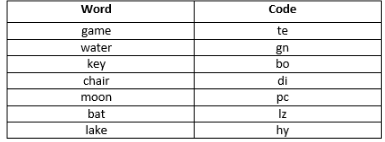Hence, option B is the correct choice.

Study the following information carefully and answer the questions which follow:
In a certain language
‘game water key chair’ is coded as ‘gn te di bo’
’chair moon bat water’ is coded as ‘pc di lz gn’
‘lake water key bat’ is coded as ‘lz bo gn hy’

RRB Group D Mock Test - 30 - Question 85

Which word among the following options is coded as ‘gn’?

Detailed Solution for RRB Group D Mock Test - 30 - Question 85

We know that, ‘game water key chair’ is coded as ‘gn te di bo’ ’chair moon bat water’ is coded as ‘pc di lz gn’ ‘lake water key bat’ is coded as ‘lz bo gn hy’ From 1st and 2nd we can say that the code for water and chair must be either gn or di. So the code for game and key must be either te or bo. From the third statement, we can say that code for lake is hy, code for key is bo and code for bat is lz. Thus we have,Hence, option D is the correct option.

Study the following information carefully and answer the questions which follow:
In a certain language
‘game water key chair’ is coded as ‘gn te di bo’
’chair moon bat water’ is coded as ‘pc di lz gn’
‘lake water key bat’ is coded as ‘lz bo gn hy’

RRB Group D Mock Test - 30 - Question 86

What is the code for the word ‘game’?

Detailed Solution for RRB Group D Mock Test - 30 - Question 86

We know that, ‘game water key chair’ is coded as ‘gn te di bo’ ’chair moon bat water’ is coded as ‘pc di lz gn’ ‘lake water key bat’ is coded as ‘lz bo gn hy’ From 1st and 2nd we can say that the code for water and chair must be either gn or di. So the code for game and key must be either te or bo. From the third statement, we can say that code for lake is hy, code for key is bo and code for bat is lz. Thus we have,Hence, option C is the correct choice.

RRB Group D Mock Test - 30 - Question 87

Choose the correct alternative from the given ones that will complete the seriesFHK, LNQ, RTW, XZC?

Detailed Solution for RRB Group D Mock Test - 30 - Question 87

F + 2 = H and H + 3 = K
K + 1 = L
L + 2 = N and N + 3 = Q
Q + 1 = R and so on.
So, C + 1 = D
D + 2 = F and F + 3 = I
Hence, option A is the correct choice.

RRB Group D Mock Test - 30 - Question 88

In each of the following question select the related numbers/words/letters from the given options.Leaf : Green : : ? : White

Detailed Solution for RRB Group D Mock Test - 30 - Question 88

Leaf is normally green in colour, at the same time paper is usually white.
Hence, option D is the right answer.

RRB Group D Mock Test - 30 - Question 89

In each of the following question select the related numbers/words/letters from the given options.USA : Dollar : : Finland : ?

Detailed Solution for RRB Group D Mock Test - 30 - Question 89

The currency of the USA is dollar and currency of Finland is Euro.
Hence, option C is the right answer.

RRB Group D Mock Test - 30 - Question 90

In each of the following question select the related numbers/words/letters from the given options.BX : FS : : GN : ?

Detailed Solution for RRB Group D Mock Test - 30 - Question 90

B + 4 = F
X - 5 = S
G + 4 =K
N - 5 = I
Hence, option B is the right answer.

RRB Group D Mock Test - 30 - Question 91

Statements
In a football match, a team won the match with score 8-2. Out of the 8 goals, 6 were scored by defenders.
Conclusions
I. More than half of the winning team consisted of defenders.
II. The winning team had better goalkeeper than the losing team.

Detailed Solution for RRB Group D Mock Test - 30 - Question 91

We know that 6 goals out of the 8 were scored by defenders. With this information, we cannot say anything about the number of defenders in the team. Thus, conclusion I does not follow.
Also, nothing can be said about which team had a better goalkeeper. Thus, conclusion II does not follow.
Hence, option C is the right answer.

RRB Group D Mock Test - 30 - Question 92

Statements
African countries will face a rise in population and at the same time depletion in the resources in the coming decade.
Conclusions
I. Developing countries are facing a similar problem today.
II. Governments of African countries will have problems in providing excellent quality of life to its citizens

Detailed Solution for RRB Group D Mock Test - 30 - Question 92

We know that there will be a rise in population and depletion of resources. So the government will face problems in providing quality life to all citizens. Thus, conclusion II follows.
But with the given information we cannot conclude anything about the present situation in developing countries. Thus, conclusion I follows.
Hence, option B is the right answer.

RRB Group D Mock Test - 30 - Question 93

Statements
State governments have appointed tourism brand ambassadors and are offering attractive tourist packages to foreign nationals.
Conclusions
I. State governments are serious about attracting more tourists.
II. State governments can expect a rise in the revenue of state if the more foreign tourists visit them.

Detailed Solution for RRB Group D Mock Test - 30 - Question 93

Since the state government are considering many initiatives to boost state tourism, we can say that they are serious about attracting more tourists. Thus, conclusion I follows.
Further, a state government would encourage more tourist with a view that it will be able to generate more revenues. Thus, conclusion II follows.
Hence, option D is the right answer.

RRB Group D Mock Test - 30 - Question 94

Select the option that is different from the other 3 options:

Detailed Solution for RRB Group D Mock Test - 30 - Question 94

Telangana, Chhattisgarh and Madhya Pradesh are land locked states. Odisha has a coastline. Therefore, option C is the right answer.

RRB Group D Mock Test - 30 - Question 95

Which of the following is an odd one out?

Detailed Solution for RRB Group D Mock Test - 30 - Question 95

Azure, Office suite and skype are products of Microsoft. Chromecast is a product of Google.
Hence, option C is the right answer.

RRB Group D Mock Test - 30 - Question 96

Which of the following is an odd one out?

Detailed Solution for RRB Group D Mock Test - 30 - Question 96

Wheat, Linseed and Barley are rabi crops while Maize is a kharif crop.
Hence, option A is the right answer.

RRB Group D Mock Test - 30 - Question 97

If, ‘+’ is coded as ‘#’, ‘*’ is coded as ‘@’, ‘-’ is coded as ‘$’, ‘/‘ is coded as ‘&’then what is the value of 14 # 27 & 3$ 4 @ 5?

Detailed Solution for RRB Group D Mock Test - 30 - Question 97

14 # 27 & 3 $4 @ 5 would mean 14 + (27/3) - 4 * 5 = 14 + 9 - 20 = 3 Hence, option D is right choice. RRB Group D Mock Test - 30 - Question 98 Suraj walks 11 km towards east and then takes a left turn to walk 12 km before taking a left turn to walk 6 km again to reach the destination. What is the shortest distance between start and end point? Detailed Solution for RRB Group D Mock Test - 30 - Question 98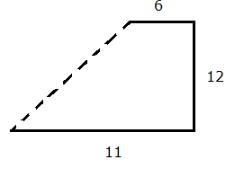The distance between final and starting point can be given by Pythagoras theorem,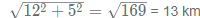Hence, option B is the right answer. RRB Group D Mock Test - 30 - Question 99 A + B means A is the son of BA * B means A is the grandson of BA$ B means A is the brother of BA ÷ B means A is the wife of BAccording to which of the following options, E is daughter in law of Q?

Detailed Solution for RRB Group D Mock Test - 30 - Question 99

In E ÷ M \$ H + Q, E is the wife of M, M is the brother of H and H is the son of Q. So, Q must be the parent of M, and thus, E is daughter in law of Q.
Hence, option B is the correct option.

RRB Group D Mock Test - 30 - Question 100

According to the following venn diagram how many bikes are electric but not red in colour?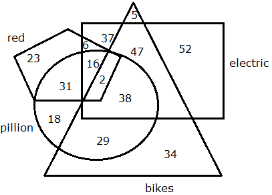Detailed Solution for RRB Group D Mock Test - 30 - Question 100

We want intersection of triangle and rectangle but avoid pentagon.
Thus required answer = 38 + 47 = 85
Hence, option D is the right choice.

## RRB (Group D) - Mock Tests & Previous Year Papers

1 docs|48 tests
 Use Code STAYHOME200 and get INR 200 additional OFF Use Coupon Code
Information about RRB Group D Mock Test - 30 Page
In this test you can find the Exam questions for RRB Group D Mock Test - 30 solved & explained in the simplest way possible. Besides giving Questions and answers for RRB Group D Mock Test - 30, EduRev gives you an ample number of Online tests for practice

1 docs|48 tests